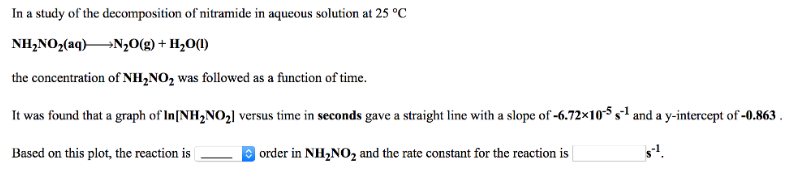# Problem: In a study of the decomposition of nitramide in aqueous solution at 25°C.NH2NO2 (aq) → N2O (g) + H2O (l) the concentration of NH2NO2 was followed as a function of time. It was found that a graph of ln[NH2NO2] versus time in seconds gave a straight line with a slope of -6.72 x 10-5 s-1 and a y-intercept of -0.863. Based on this plot, the reaction is ________ order in NH2NO2 and the rate constant for the reaction is _____________ s-1.

###### FREE Expert Solution
93% (193 ratings)###### Problem Details

In a study of the decomposition of nitramide in aqueous solution at 25°C.

NH2NO(aq) → N2O (g) + H2O (l)

the concentration of NH2NO2 was followed as a function of time. It was found that a graph of ln[NH2NO2] versus time in seconds gave a straight line with a slope of -6.72 x 10-5 s-1 and a y-intercept of -0.863.

Based on this plot, the reaction is ________ order in NH2NO2 and the rate constant for the reaction is _____________ s-1.